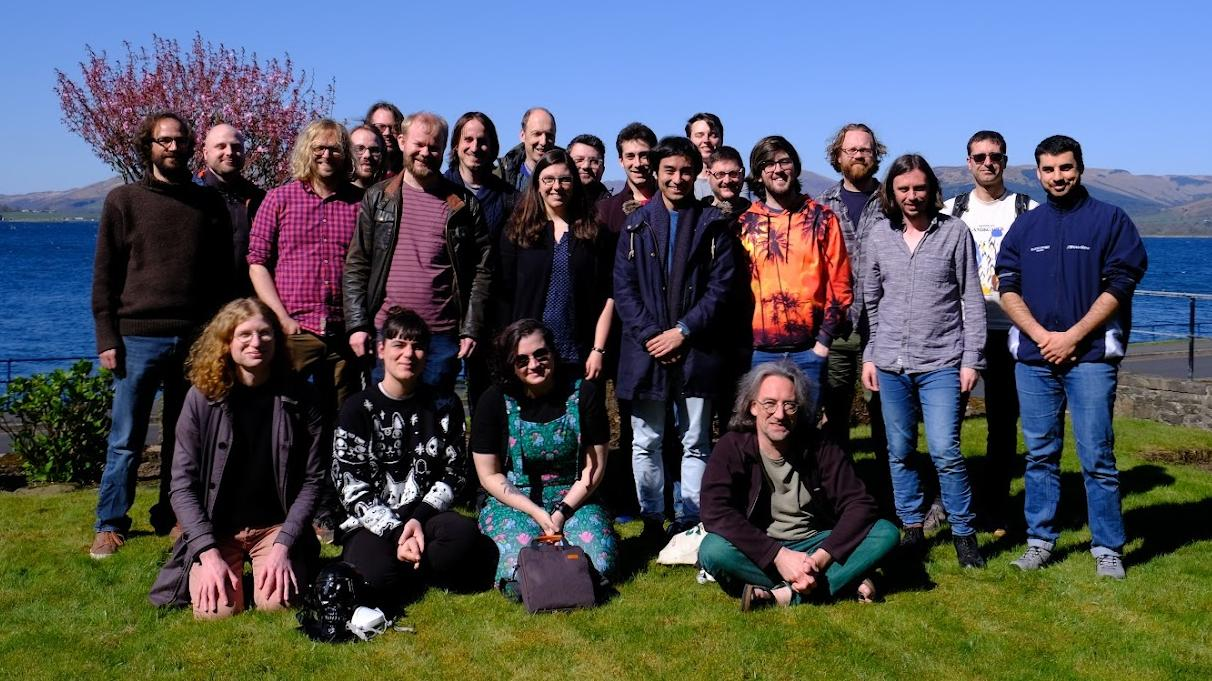## Mathematically Structured Programming Group## Research Themes

Our vision is to use mathematics to understand the nature of computation, and to turn that understanding into the next generation of programming languages.

We see the mathematical foundations of computation and programming as inextricably linked. We study one so as to develop the other. This reflects the symbiotic relationship between mathematics, programming, and the design of programming languages — any attempt to sever this connection will diminish each component.

To achieve these research goals we use ideas from the following disciplines:

Functional Programming and Type Theory
What does the future of programming languages look like? How does one take the logical structure of computation and turn it into a programming abstraction? Type theory allows us to do this by providing a language at an intermediate level of abstraction between a programming language and its logical foundations. Indeed, type theory could be said to be the ideas factory for programming languages.
Logic
Why is functional programming successful as a model of computation? The answer lies in its genesis as a clean implementation of the logical structure of computation. Thus we develop functional programming by studying the logical structure of computation.We also study logical and algebraic techniques in their own right, using modal logics and quantitative algebras to study dynamic and approximate properties of systems.
Category Theory
How does one understand structure abstractly? How can one build theories that systematically build complex systems by composing descriptions of simpler ones? One uses category theory — that's how! Ideas such as monads and initial algebra semantics attest to the deep contribution that category theory has made to computation.